# Class 8 RD Sharma Solutions – Chapter 20 Area Of Trapezium And Polygon- Exercise 20.3

• Last Updated : 07 Nov, 2021

### Question 1. Find the area of the pentagon shown in figure below, if AD = 10 cm, AG = 8 cm, AH = 6 cm, AF = 5 cm, BF = 5 cm, CG = 7 cm and EH = 3 cm.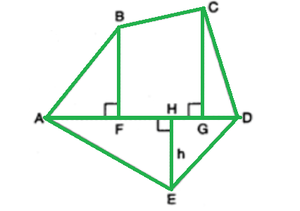Solution:

Given:

AD = 10 cm, AG = 8 cm, AH = 6 cm, AF = 5 cm BF = 5 cm, CG = 7 cm, EH = 3 cm

From given data,

FG = AG – AF = 8 – 5 = 3 cm

And,

GD = AD – AG = 10 – 8 = 2 cm

From given figure:

Area of Pentagon = (Area of triangle AFB) + (Area of trapezium FBCG) +

(Area of triangle CGD) + (Area of triangle ADE)

= (0.5 x AF x BF) + [0.5 x (BF + CG) x (FG)] + (0.5 x GD x CG) + (1/2 x AD x EH).

= (0.5 x 5 x 5) + [0.5 x (5 + 7)x (3) + (0.5 x 2 x 7) + (0.5 x 10 x 3)

= 12.5 + 18 + 7 + 15 = 52.5 cm

### Questions 2. Find the area enclosed by each of the following fig(Fig. (i)-(iii)J as the sum of the areas of a rectangle and a trapezium.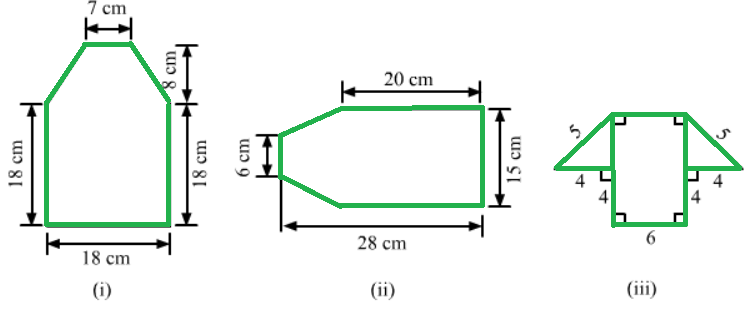Solution:

(i) Figure can be divided into 2 parts a square and a trapezium as shown:

Area = (Area of square) + (Area of trapezium)

= (side)2 + (0.5 x (sum of parallel sides) x height

= (18 x 18) + 0.5 x (18 + 7) × (8)

= 324+100

= 424 cm2

(ii) Figure can be divided into 2 parts a rectangle and a trapezium as shown:

From the figure:

Height of trapezium = 28 – 20 = 8 cm

Area = (Area of rectangle) + (Area of trapezium)

= (length x breadth) + (0.5 x (sum of parallel sides) x height)

= (20 x 15) + [0.5 x (15 + 6) × (8)]

= 300 + 84

= 384 cm2

(iii) Figure can be divided into 2 parts one trapezium and one rectangle:

Pythagoras theorem in one of the right angle triangle:

52 = 42 + (base)2

base2 = 25 – 16

base = √9 = 3 cm

Therefore,

The height of trapezium = 3 cm

One side of trapezium = 6 + 4 + 6 = 14 cm

Area = (Area of rectangle) + (Area of trapezium)

= (length x breadth) + (0.5 x (sum of parallel sides) x height)

= (6 x 4) + (0.5 x (14 + 6) x (3))

= 24 + 30

= 54 cm2

### Question 3. There is a pentagonal shaped park as shown in Fig. Jyoti and Kavita divided it in two different ways.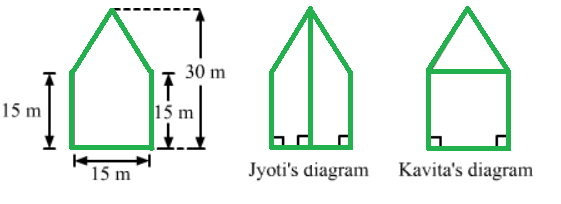### Find the area of this park using both ways. Can you suggest some another way of finding its area?

Solution:

Jyoti and Kavita divided the park in two different ways.

(i) Jyoti divided the park into two equal trapeziums:

From the figure the park divided into equal trapezium having height 7.5 m and sides 30 m and 15 m

Area of the park = 2 x (Area of a trapezium)

= 2 x (0.5 x (sum of parallel sides) x height)

= 2 x (0.5 x (30 + 15) x (7.5))

= 337.5 m2

(ii) Kavita divided the park into a rectangle and a triangle:

From diagram,

The height of the triangle = 30 – 15 = 15 m

Area of the park = (Area of square) + (Area of triangle)

= (15 x 15) + (0.5 x 15 x 15)

= 225 + 112.5

= 337.5 m2

### Question 4. Find the area of the following polygon, if AL = 10 cm, AM = 20 cm, AN = 50 cm, AO = 60 cm and AD = 90 cm.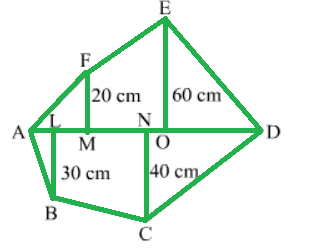Solution:

Given:

AL = 10 cm, AM = 20 cm, AN = 50 cm

AO = 60 cm, AD = 90 cm

From given data,

MO = AO – AM = 60 – 20 = 40 cm

OD = AD – A0 = 90 – 60 = 30 cm

ND = AD – AN = 90 – 50 = 40 cm

LN = AN – AL = 50 – 10 = 40 cm

Area of Polygon = (Area of triangle AMF) + (Area of trapezium MOEF) +

(Area of triangle DNC) + (Area of trapezium NLBC) +

(Area of triangle ALB)

= (0.5 x AM x MF) + [0.5 x (MF + OE) x OM] + (0.5 x OD x OE) +

(0.5 x DN x NC) + [0.5 x (LB + NC) x NL] + (0.5 x AL x LB)

= (0.5 x 20 x 20) + [0.5 x (20 + 60) x (40)] +(0.5 x 30 x 60) +

(0.5 x 40 x 40) +[0.5 x (30 + 40) x (40)] + (0.5 x 10 x 30)

= 200 + 1600 + 900 + 800 + 1400 +150 = 5050 cm2

### Question 5. Find the area of the following regular hexagon.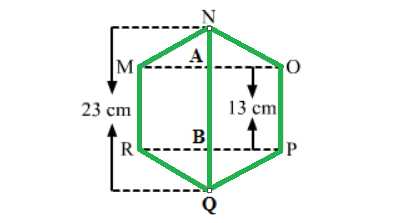Solution:

As it is a regular Hexagon so all sides are 13 cm and AN = BQ

From the figure,

As the diagonal QN is 23 cm,

QB + BA + AN = QN

AN + 13 + AN = 23

2AN = 23 – 13 = 10

AN = 5 cm

Hence, AN = BQ = 5 cm

Applying Pythagoras theorem in triangle MAN:

MN2 = AN2 + AM2

169 = 25 + AM2

AM² = 169 – 25

AM = √144

AM = 12cm

From figure,

OM = RP = 2 × AM = 2 x 12 = 24 cm

This Hexagon can be divided into 3 parts 2 triangles and one rectangle therefore,

Area of the regular hexagon = (area of triangle MON) + (area of rectangle MOPR) +

(area of triangle RPQ)

= (0.5 x OM x AN) + (RP X PO) + (0.5 x RP x BQ)

= (0.5 x 24 x 5) + (24 x 13) + (0.5 x 24 x 5)

= 60 + 312 + 60

= 432 cm2

My Personal Notes arrow_drop_up